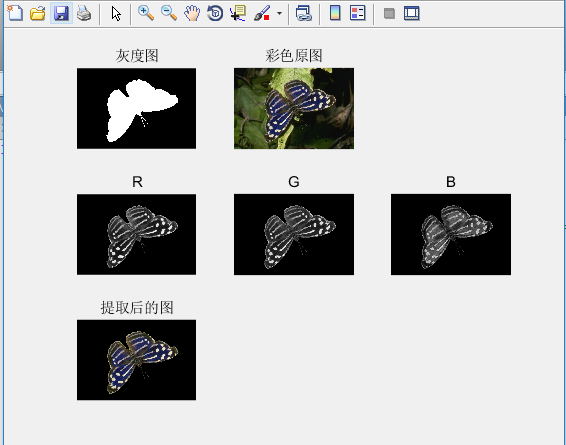# 方法三：

#### 代码如下：

clear,clc,close all;
a1=im2bw(a);            %灰度图转为二值图（矩阵元素变为0和1）
a1=uint8(a1);           %由于二值图元素的类型都是 logical型（逻辑型），在进行运算处理时不符合要求，所以转为 uint8
%由于b是原图，有三个通道，分别为R通道、G通道、B通道。每一个通道对应一个二维矩阵

R=b(:,:,1);             %分别提取三个通道（也就是三个二维矩阵）
G=b(:,:,2);
B=b(:,:,3);
R1=R.*a1;               %各个通道的矩阵乘以二值图，中心的蝴蝶矩阵数值不变，背景变为0
G1=G.*a1;
B1=B.*a1;
b1(:,:,1)=R1;           %三个通道叠加，组成一个三维的RGB图
b1(:,:,2)=G1;
b1(:,:,3)=B1;
subplot(331),imshow(a),title('灰度图');
subplot(332),imshow(b),title('彩色原图');
subplot(334),imshow(R1),title('R');
subplot(335),imshow(G1),title('G');
subplot(336),imshow(B1),title('B');
subplot(337),imshow(b1),title('提取后的图');



#### 运行截图：